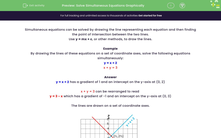# Solve Simultaneous Equations Graphically

In this worksheet, students will practise solving simple simultaneous equations graphically by finding the point of intersection on an x-y graph.Key stage:  KS 3

Curriculum topic:   Algebra

Curriculum subtopic:   Understand Expressions, Equations, Inequalities, Terms and Factors

Difficulty level:#### Worksheet Overview

Simultaneous equations can be solved by drawing the line representing each equation and then finding the point of intersection between the two lines.

Use y = mx + c, or other methods, to draw the lines.

Example

By drawing the lines of these equations on a set of coordinate axes, solve the following equations simultaneously:

y = x + 2

x + y = 3

y = x + 2 has a gradient of 1 and an intercept on the y-axis at (0, 2)

x + y = 3 can be rearranged to read

y = 3 - x which has a gradient of -1 and an intercept on the y-axis at (0, 3)

The lines are drawn on a set of coordinate axes.The point of intersection is (½, 2½) and this is the only point at which both equations are satisfied.

So the solution to the simultaneous equations is x = ½, y = 2½.

### What is EdPlace?

We're your National Curriculum aligned online education content provider helping each child succeed in English, maths and science from year 1 to GCSE. With an EdPlace account you’ll be able to track and measure progress, helping each child achieve their best. We build confidence and attainment by personalising each child’s learning at a level that suits them.

Get started